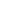# Calculator of forex volatilityVolatility is a statistical measure to gauge fluctuations on Forex. This term reflects a size of changes in a security’s value for a certain time interval. Besides, it is an important financial barometer, which determines an amount of risk for a particular deal. For instance, currency pairs with a low volatility are less risky as their values do not fluctuate dramatically. On the contrary, currency pairs with a high volatility require a well-grounded trading strategy.

The average volatility calculator is created to assess a price volatility of a particular currency pair for a certain period. A trader can estimate volatility of major, exotic, and cross currency pairs. A calculation is based on an intraday change in pips and percent according to a certain time frame from 1 to 52 weeks. The volatility calculator helps traders evaluate the degree of their investment risks.

Traders should be aware of an average volatility for every currency pair. The calculator enables traders to measure a standard volatility for different time frames as well as deviations from an average level. Thus, traders can recognize a favorable period for trading. Importantly, too “old” data can be unimportant to make a forecast.

The average true range (ATR) indicator is used to measure market volatility. Please follow the formula below:
Formula: Variation = Average (Higher — Lower)
Interpretation: Variation equals an average value of a difference between the highest and lowest prices of a currency pair over a particular period.

Market quotes for calculations are provided by InstaForex.

Currency Pairs to Show

To calculate volatility enter the number of weeksMessage
error_textابدأ التداول بدون مخاطر واستثمارات
مع "بونص ستارت أب" الجديد البالغ قدره \$1000 دولاراحصل على بونص
55%
من إنستافوركس
على كل إيداعاكسب ما يصل الى
\$50000
لتوجيه دعوة إلى الأصدقاء للحصول البونص البدائي من إنستافوركس
ليست أي استثمارات مطلوبة!